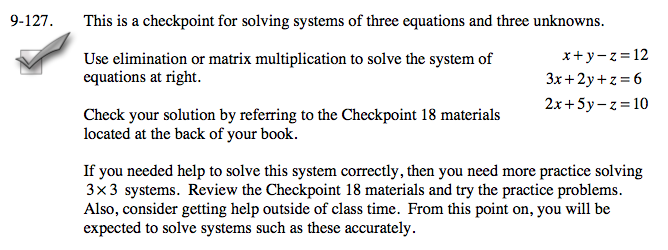Home > A2C > Chapter 9 > Lesson 9.3.1 > Problem9-127

9-127.This is a checkpoint for solving systems of three equations and three unknowns.Use elimination or matrix multiplication to solve the system of equations below.

x + yz = 12
3x + 2y + z = 6
2x + 5yz = 10

Check your solution by referring to the Checkpoint 18 materials located at the back of your book.
If you needed help to solve this system correctly, then you need more practice solving 3x3 systems. Review the Checkpoint 18 materials and try the practice problems. Also, consider getting help outside of class time. From this point on, you will be expected to solve systems such as these accurately. Homework Help ✎Answers and extra practice are located in the back of your printed textbook or in the Reference Tab of your eBook.
If you have an eBook for A2C , login and then click the following link: Checkpoint 18 Answers and Practice